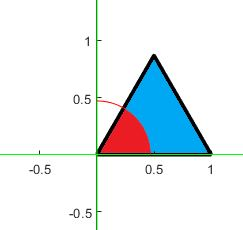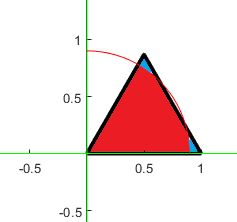# Problem 49938. Splitting Triangle - Problem the third

Consider an equilateral triangle sitting in Quadrant I as depicted in an example below:This triangle is to be split into two regions (e.g., red and blue). Given the ratio between the two regions and the side of the equilateral triangle, determine the radius of the circle defining the red region. The ratio between the regions (red to blue) is presented through the first two entries in the input. For example, if the ratio is 3 to 5, then these two numbers will be the first two entries in the input. The last entry is the side of the equilateral triangle. Please also consider the following possible configuration:### Solution Stats

41.67% Correct | 58.33% Incorrect
Last Solution submitted on Jun 10, 2023

### Community Treasure Hunt

Find the treasures in MATLAB Central and discover how the community can help you!

Start Hunting!